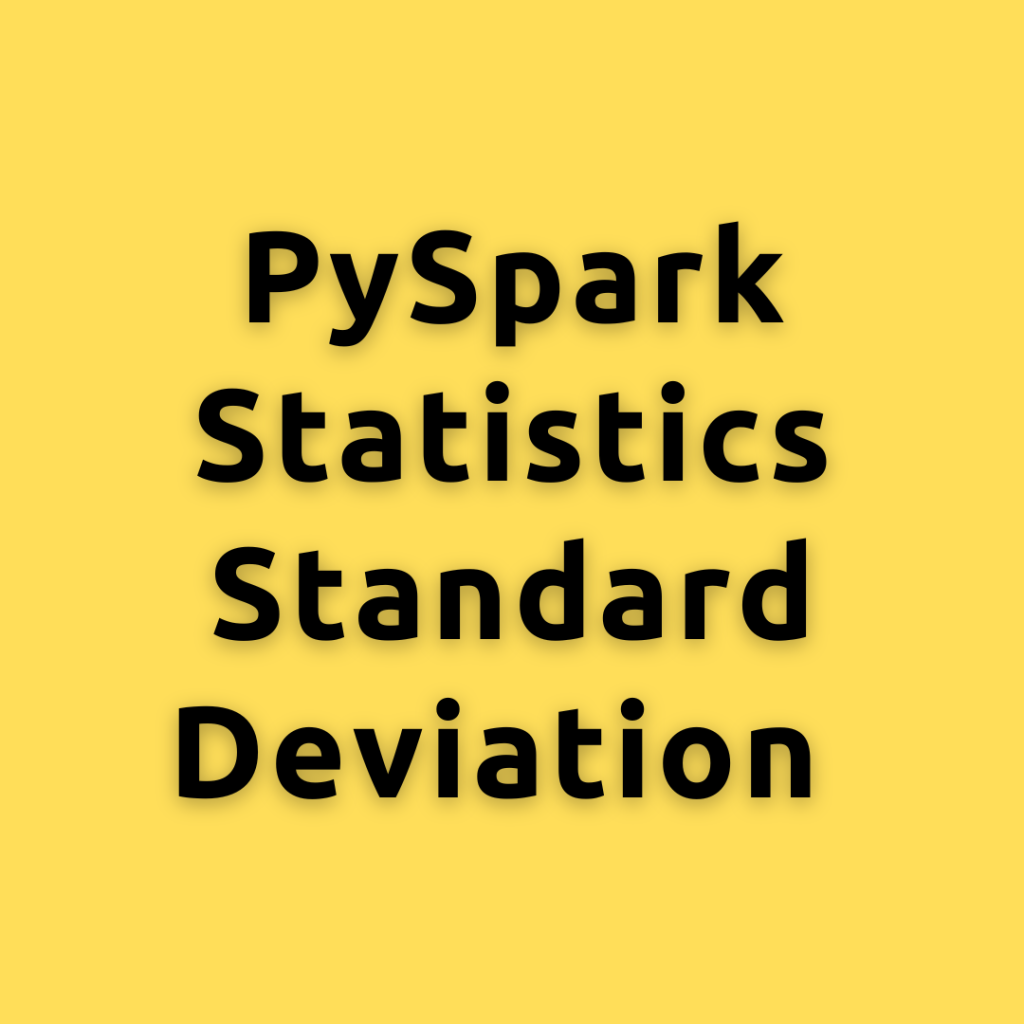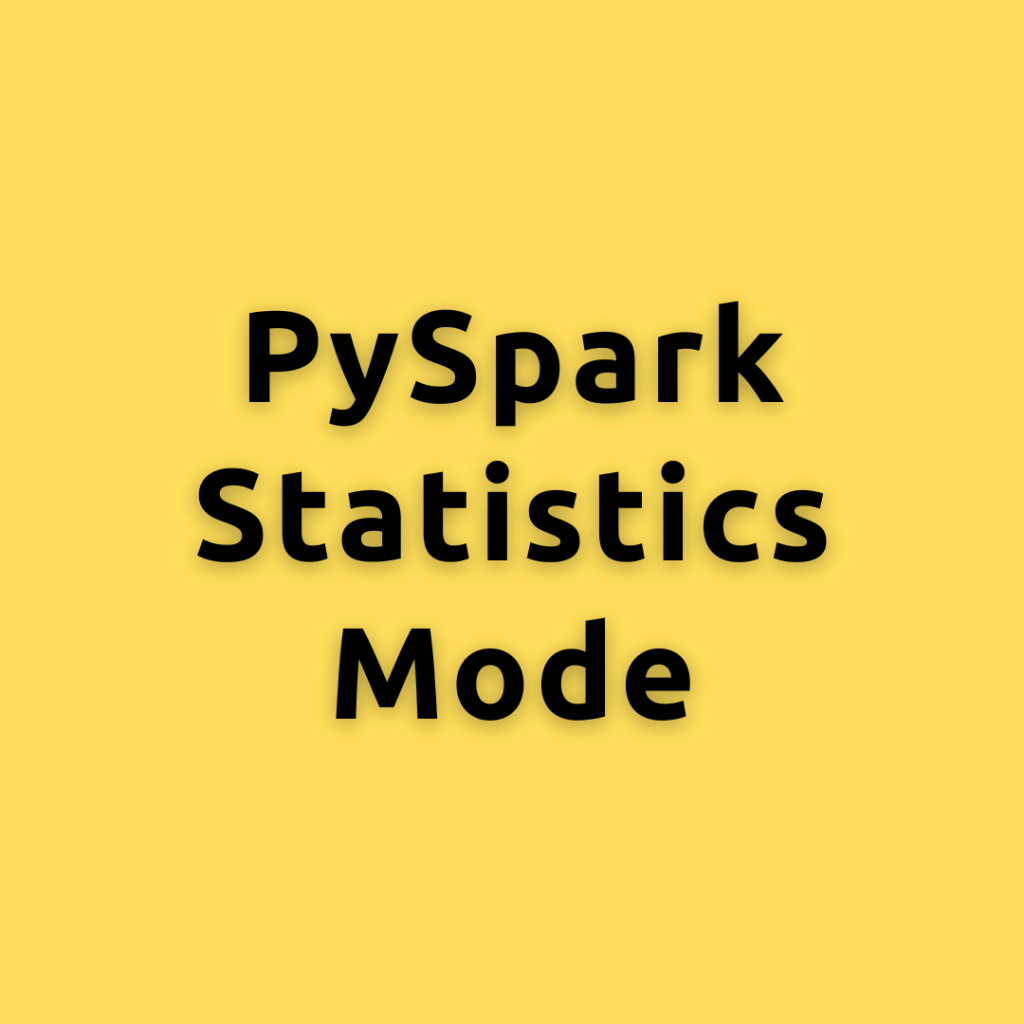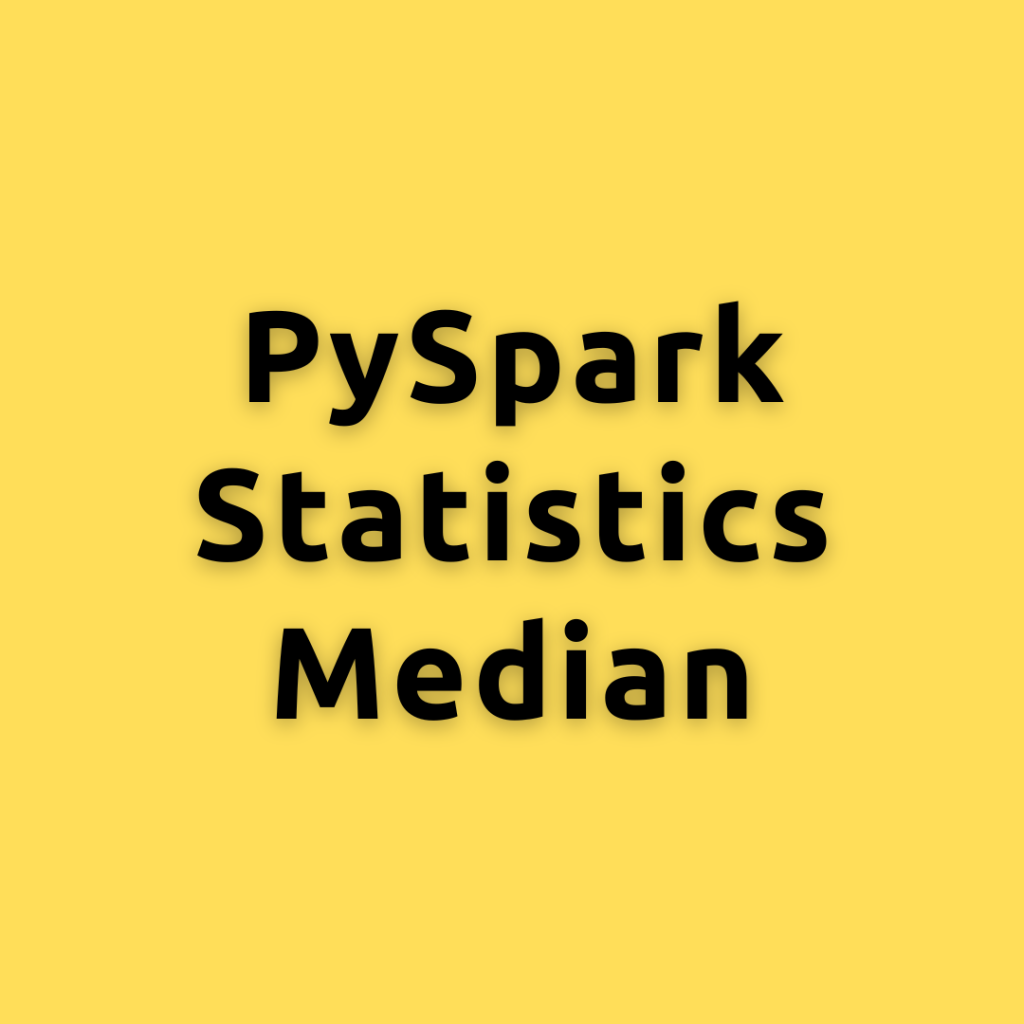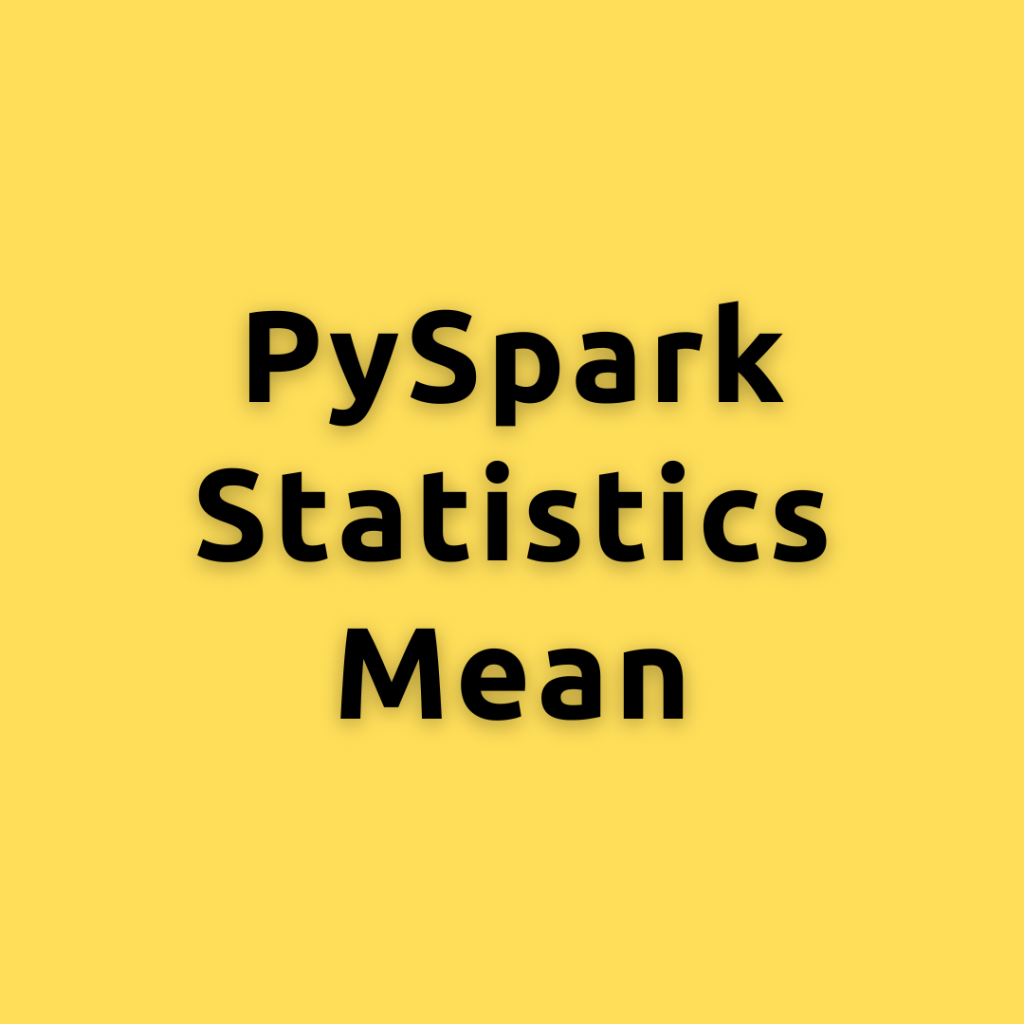Drop a Query

# May 2, 2023## PySpark Statistics Standard Deviation – Calculating the Standard Deviation in PySpark a Comprehensive Guide for Everyone

Lets dive into the concept of Standard Deviation, its importance in statistics and machine learning, and explore different ways to calculate it using PySpark How to Calcualte Standard Deviation? Standard Deviation is a measure that quantifies the amount of variation or dispersion in a set of data values. It helps in understanding how far individual …## PySpark Statistics Mode – Calculating the Mode in PySpark a Comprehensive Guide for Everyone

Lets explore different ways of calculating the Mode using PySpark, helping you become an expert Mode is the value that appears most frequently in a dataset. It is a measure of central tendency, similar to mean and median, but focuses on the most common value(s) in the data. Mode can be applied to both numerical …## PySpark Statistics Median – Calculating the Median in PySpark a Comprehensive Guide for Everyone

Lets explore different ways of calculating the Median using PySpark, helping you become an expert As data continues to grow exponentially, efficient data processing becomes critical for extracting meaningful insights. PySpark, an Apache Spark library, enables large-scale data processing in Python. How to Calcualte Median? The median is a measure of central tendency that represents …## PySpark Statistics Mean – Calculating the Mean Using PySpark a Comprehensive Guide for Everyone

Lets explore different ways of calculating the mean using PySpark, helping you become an expert in no time As data continues to grow exponentially, efficient data processing becomes critical for extracting meaningful insights. PySpark, an Apache Spark library, enables large-scale data processing in Python. Concept of Mean: The mean, also known as the average, is …Course Preview

## Machine Learning A-Z™: Hands-On Python & R In Data Science

### Free Sample Videos:#### Machine Learning A-Z™: Hands-On Python & R In Data Science#### Machine Learning A-Z™: Hands-On Python & R In Data Science#### Machine Learning A-Z™: Hands-On Python & R In Data Science#### Machine Learning A-Z™: Hands-On Python & R In Data Science